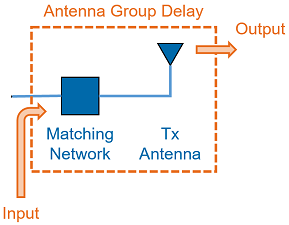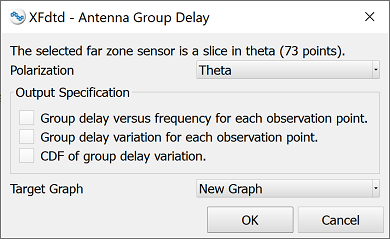# Antenna Group Delay Script | XFdtd

Compute and plot the single-antenna result.The Antenna Group Delay script allows users to compute group delay for a single-antenna simulation. Antenna group delay is impacted by the antenna's input impedance and the observation angles determined by a far-zone sensor. For a single far zone observation point, antenna group delay versus frequency, $\tau(f)$, is computed. Given $\tau(f)$ at a single point, the group delay variation, $\delta$, is $\tau(f)_{max} - \tau(f)_{min}$. For N observation points, there are N variation floating point numbers. A cumulative distribution function, CDF, is determined using the N variations.

The script's output options include generating graphs of $\tau(f)$ for each observation point in the far zone sensor, $\delta$ for each observation point when the far zone sensor is a slice in theta or phi, and the CDF of $\delta$.

## Prerequisites

In order for the script to function properly, users must complete the following steps as part of the project setup workflow for antenna analysis:

• Add a steady-state far zone sensor with observation points where group delay is computed.
• Request multiple steady-state frequencies when creating the FDTD simulation. For increased efficiency, users can refer to XF's syntax for specifying frequency sets. For example, [2 GHz : 4 GHz : 0.5 GHz].

## Workflow

Use the antenna group delay macro by following these steps:

2. Either place the file in your Macros Menu directory, or right-click on Scripts in the Project Tree and choose Import Scripts.

A result must be selected in order for the script to operate.

1. Select a far zone sensor result, such as gain, in the bottom portion of the Results browser.
2. Execute the script by selecting it in the Macros Menu, or right-clicking on the imported script in the Project Tree and choosing Execute.Executing the script opens a user-input window. The available outputs depend on whether the selected far zone sensor contains a single observation point, multiple points contained in a single slice in theta or phi, or multiple points in both axes.

1. Select the desired Polarization.
2. Select the output results of interest.
3. Select the desired Target Graph.
4. Click OK.

The script reads the required data off disk, computes antenna group delay for the specified observation angles, and outputs $\tau(f)$, $\delta$, or a CDF of $\delta$ to a graph.